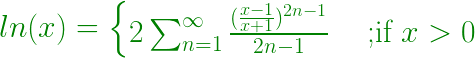Related Articles
Find the ln(X) and log10X with the help of expansion
• Last Updated : 28 Aug, 2019

Given a positive number x, the task is to find the natural log (ln) and log to the base 10 (log10) of this number with the help of expansion.

Example:

Input: x = 5
Output: ln 5.000 = 1.609
log10 5.000 = 0.699

Input: x = 10
Output: ln 10.000 = 2.303
log10 10.000 = 1.000


## Recommended: Please try your approach on {IDE} first, before moving on to the solution.

Approach:

1. The expansion of natural logarithm of x (ln x) is:

2. Therefore this series can be summed up as:3. Hence a function can be made to evaluate the nth term of the sequence for 1 &leq; x &leq; n
4. Now to calculate log10 x, below formula can be used:

Below is the implementation of the above approach:

## C++

 // CPP code to Find the ln x and// log10 x with the help of expansion  #include #include #include   using namespace std;  // Function to calculate ln x using expansiondouble calculateLnx(double n){      double num, mul, cal, sum = 0;    num = (n - 1) / (n + 1);      // terminating value of the loop    // can be increased to improve the precision    for (int i = 1; i <= 1000; i++) {        mul = (2 * i) - 1;        cal = pow(num, mul);        cal = cal / mul;        sum = sum + cal;    }    sum = 2 * sum;    return sum;}  // Function to calculate log10 xdouble calculateLogx(double lnx){    return (lnx / 2.303);}  // Driver Codeint main(){      double lnx, logx, n = 5;    lnx = calculateLnx(n);    logx = calculateLogx(lnx);      // setprecision(3) is used to display    // the output up to 3 decimal places      cout << fixed << setprecision(3)         << "ln " << n << " = "         << lnx << endl;    cout << fixed << setprecision(3)         << "log10 " << n << " = "         << logx << endl;}

## Java

 // Java code to Find the ln x and// log10 x with the help of expansionimport java.io.*;  class GFG {      // Function to calculate ln x using expansionstatic double calculateLnx(double n){    double num, mul, cal, sum = 0;    num = (n - 1) / (n + 1);      // terminating value of the loop    // can be increased to improve the precision    for (int i = 1; i <= 1000; i++)     {        mul = (2 * i) - 1;        cal = Math.pow(num, mul);        cal = cal / mul;        sum = sum + cal;    }    sum = 2 * sum;    return sum;}  // Function to calculate log10 xstatic double calculateLogx(double lnx){    return (lnx / 2.303);}  // Driver Codepublic static void main (String[] args) {    double lnx, logx, n = 5;    lnx = calculateLnx(n);    logx = calculateLogx(lnx);          // setprecision(3) is used to display    // the output up to 3 decimal places          System.out.println ("ln " + n + " = " + lnx );    System.out.println ("log10 " + n + " = "+ logx );}}  // This code is contributed by ajit

## Python3

 # Python 3 code to Find the ln x and# log10 x with the help of expansion# Function to calculate ln x using expansionfrom math import powdef calculateLnx(n):    sum = 0    num = (n - 1) / (n + 1)      # terminating value of the loop    # can be increased to improve the precision    for i in range(1, 1001, 1):        mul = (2 * i) - 1        cal = pow(num, mul)        cal = cal / mul        sum = sum + cal      sum = 2 * sum    return sum  # Function to calculate log10 xdef calculateLogx(lnx):    return (lnx / 2.303)  # Driver Codeif __name__ == '__main__':    n = 5    lnx = calculateLnx(n)    logx = calculateLogx(lnx)      # setprecision(3) is used to display    # the output up to 3 decimal places      print("ln", "{0:.3f}".format(n),            "=", "{0:.3f}".format(lnx))    print("log10", "{0:.3f}".format(n),               "=", "{0:.3f}".format(logx))      # This code is contributed by# Surendra_Gangwar

## C#

 // C# code to Find the ln x and// log10 x with the help of expansionusing System;      class GFG {      // Function to calculate ln x using expansionstatic double calculateLnx(double n){    double num, mul, cal, sum = 0;    num = (n - 1) / (n + 1);      // terminating value of the loop    // can be increased to improve the precision    for (int i = 1; i <= 1000; i++)     {        mul = (2 * i) - 1;        cal = Math.Pow(num, mul);        cal = cal / mul;        sum = sum + cal;    }    sum = 2 * sum;    return sum;}  // Function to calculate log10 xstatic double calculateLogx(double lnx){    return (lnx / 2.303);}  // Driver Codepublic static void Main (String[] args) {    double lnx, logx, n = 5;    lnx = calculateLnx(n);    logx = calculateLogx(lnx);          // setprecision(3) is used to display    // the output up to 3 decimal places          Console.WriteLine("ln " + n + " = " + lnx );    Console.WriteLine("log10 " + n + " = "+ logx );}}  // This code is contributed by Princi Singh
Output:
ln 5.000 = 1.609
log10 5.000 = 0.699


Attention reader! Don’t stop learning now. Get hold of all the important mathematical concepts for competitive programming with the Essential Maths for CP Course at a student-friendly price. To complete your preparation from learning a language to DS Algo and many more,  please refer Complete Interview Preparation Course.

My Personal Notes arrow_drop_up